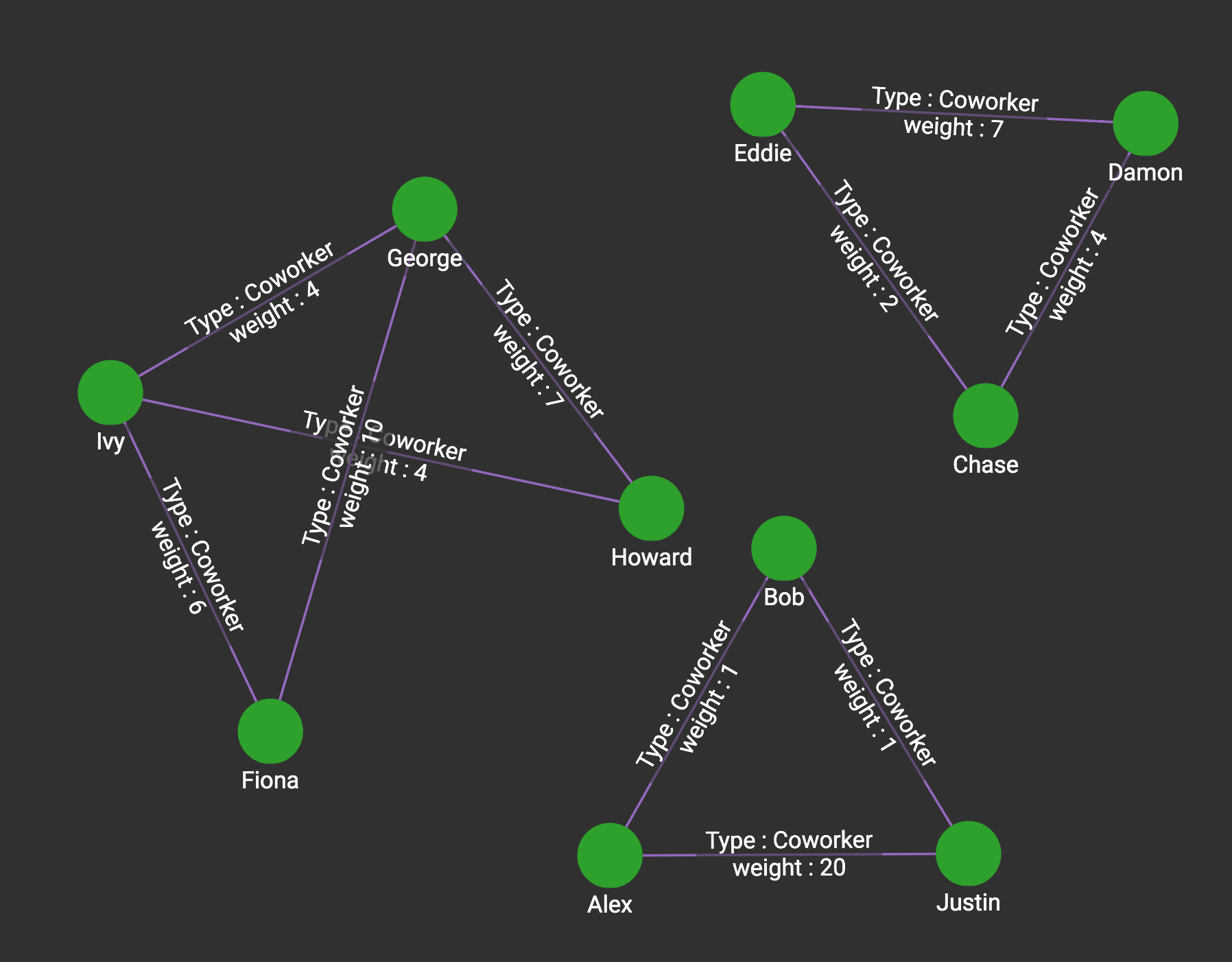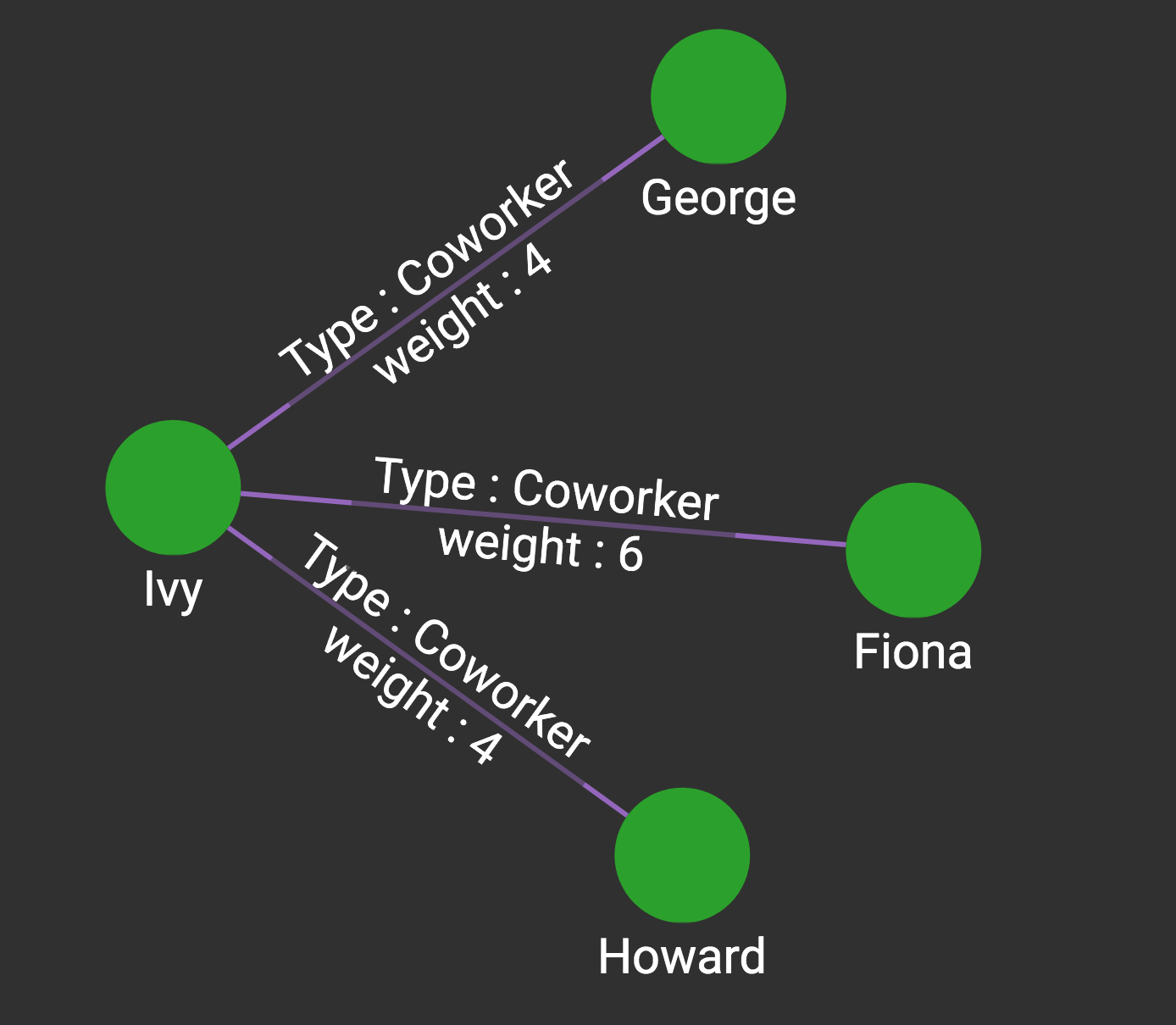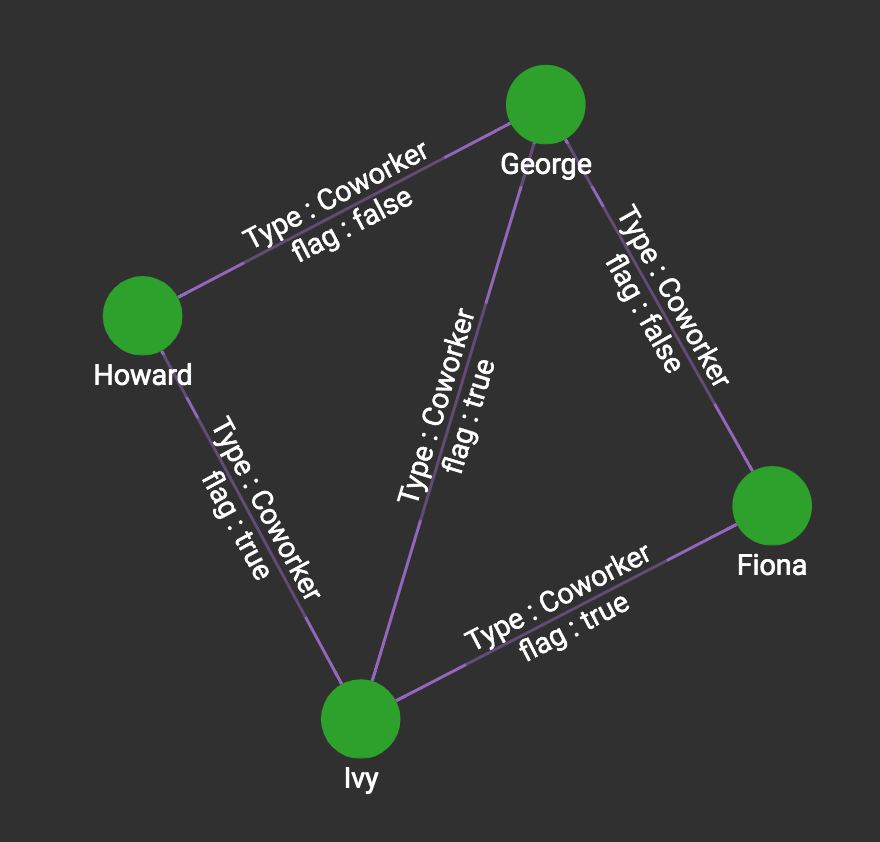# Minimum Spanning Tree

Given an undirected and connected graph, a minimum spanning tree is a set of edges that can connect all the vertices in the graph with the minimal sum of edge weights. The library implements a parallel version of Prim’s algorithm:

1. Start with a set A = { a single vertex seed }

2. For all vertices in A, select a vertex y such that

1. y is not in A, and

2. There is an edge from y to a vertex x in A, and

3. The weight of the edge e(x,y) is the smallest among all eligible pairs (x,y).

3. Add y to A, and add the edge (x,y) to MST.

4. Repeat steps 2 and 3 until A has all vertices in the graph.

If the user specifies a source vertex, this will be used as the seed. Otherwise, the algorithm will select a random seed vertex.

 If the graph contains multiple components (i.e., some vertices are disconnected from the rest of the graph, then the algorithm will span only the component of the seed vertex. If you do not have a preferred vertex, and the graph might have more than one component, then you should use the Minimum Spanning Forest (MDF) algorithm instead.

## Specifications

``````tg_mst (VERTEX opt_source, SET<STRING> v_type, SET<STRING> e_type,
STRIN wt_attr, STRING wt_type, INT max_iter = -1,
BOOL print_accum = TRUE, STRING result_attr = "", STRING file_path = "")``````
Characteristic Value

Result

Computes a minimum spanning tree. If the JSON or file output selected, the output is the set of edges that form the MST. If the result_attr option is selected, the edges which are part of the MST are tagged True; other edges are tagged False.

Input Parameters

• `VERTEX opt_source`: ID of a source vertex (optional)

• `SET<STRING> v_type`: Names of vertex types to use

• `SET<STRING> e_type`: Names of edge types to use

• `STRING wt_attr`: Name of edge weight attribute

• `STRING wt_type`: Data type of edge weight attribute: "INT", "FLOAT", or "DOUBLE"

• `INT max_iter`: Maximum of edges to include. If less than (V-1), then the result is not a true spanning tree.

• `BOOL print_accum`: If True, output JSON to standard output

• `STRING result_attr`: If not empty, store result values (BOOL) to this attribute

• `STRING file_path`: If not empty, write output to this file.

Result Size

V - 1 = number of vertices - 1

Time Complexity

O(V^2)

Graph Types

Undirected edges and connected

## Example

In the graph `social10`, we consider only the undirected Coworker edges.This graph has 3 components. Minimum Spanning Tree finds a tree for one component, so which component it will work on depends on what vertex we give as the starting point. If we select Fiona, George, Howard, or Ivy as the start vertex, then it works on the 4-vertex component on the left. You can start from any vertex in the component and get the same or an equivalent MST result.

The figure below shows the result of

``````# Use _ for default values
RUN QUERY mst(("Ivy", "Person"), ["Person"], ["Coworker"] "weight", "INT",
_, _, _, _)``````

Note that the value for the one vertex is `("Ivy", "Person")`. In GSQL, this 2-tuple format which explicitly gives the vertex type is used when the query is written to accept a vertex of any type.File output:

``````From,To,Weight
Ivy,Fiona,6
Ivy,Howard,4
Ivy,George,4``````

The attribute version requires a boolean attribute on the edge, and it will assign the attribute to "true" if that edge is selected in the MST: